Courses

# Test: Data Handling

## 10 Questions MCQ Test Mathematics (Maths) Class 6 | Test: Data Handling

Description
This mock test of Test: Data Handling for Class 6 helps you for every Class 6 entrance exam. This contains 10 Multiple Choice Questions for Class 6 Test: Data Handling (mcq) to study with solutions a complete question bank. The solved questions answers in this Test: Data Handling quiz give you a good mix of easy questions and tough questions. Class 6 students definitely take this Test: Data Handling exercise for a better result in the exam. You can find other Test: Data Handling extra questions, long questions & short questions for Class 6 on EduRev as well by searching above.
QUESTION: 1

### The following pictograph shows the number of absentees in a class of 30 students during the previous week. Which day had full attendance?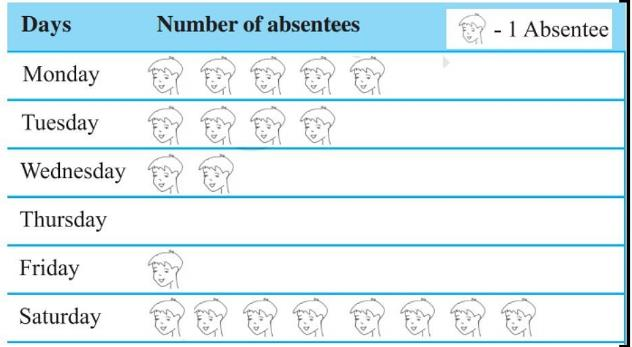Solution:

Since the day Thursday has no absentee, therefore in that day all were present and had full attendance.

QUESTION: 2

### The following pictograph shows the number of absentees in a class of 30 students during the previous week. What was the total number of absentees on Tuesday?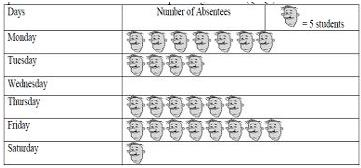Solution:

One image means 5 students and there are 4 images like these on Tuesday.

Therefore, 5 x 4 = 20

QUESTION: 3

### The following pictograph shows the number of absentees in a class of 30 students during the previous week. On which day 30 students were absent?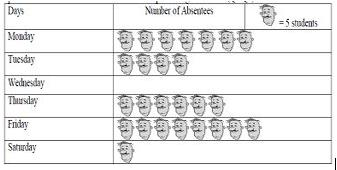Solution:

Here, one face stands for 5 students and on Thursday the pictograph shows 6 faces that mean 5 * 6  = 30

QUESTION: 4

The colours of fridges preferred by people living in a locality are shown by the following pictograph. How many people liked red colour?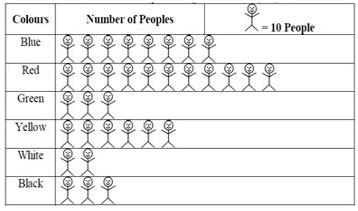Solution:

As given, 1 image  =  10
And 11 images are there for the colour Red.
Therefore,  11 * 10 = 110

QUESTION: 5

The following pictograph shows the number of absentees in a class of 30 students during the previous week. On which day were the maximum number of students absent?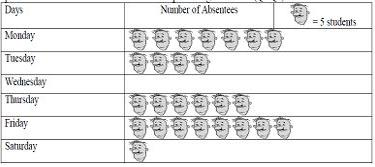Solution:

Friday is the correct ✔ answer.
As it has the most number of face images i.e. 8

QUESTION: 6

The colours of fridges preferred by people living in a locality are shown by the following pictograph. Find the number of people preferring blue colour.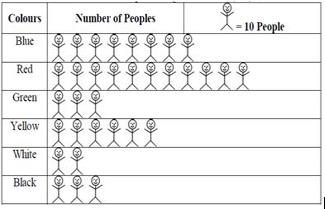Solution:

As we can see, 1 person = 10 people,
so the blue color represents 8 people.
Therefore, 8 people
=  8 * 10 ⇒ 80

QUESTION: 7

The following pictograph shows the number of absentees in a class of 30 students during the previous week. What was the total number of absentees in that week?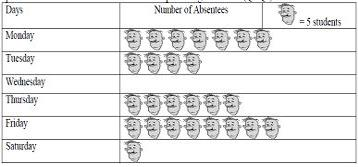Solution:

Each face image counts as 5 people. There are 26 face images in total.
Therefore,  26 x 5 = 130

QUESTION: 8

The following pictograph shows the number of absentees in a class of 60 students during the previous week. On which day 5 students were absent?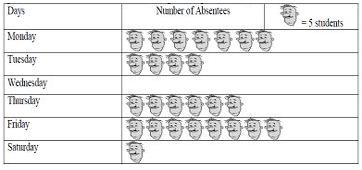Solution:

On Saturday, 5 students were absent.

QUESTION: 9

The colours of fridges preferred by people living in a locality are shown by the following pictograph. Which colour preferred most?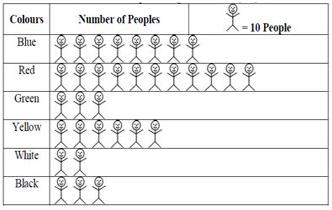Solution:

It can be observed that there are larger number of people in the red color representation. Hence, Red colour is preferred most.

QUESTION: 10

The colours of fridges preferred by people living in a locality are shown by the following pictograph. Which colour preferred least?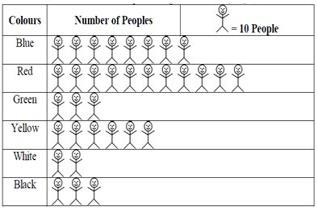Solution: